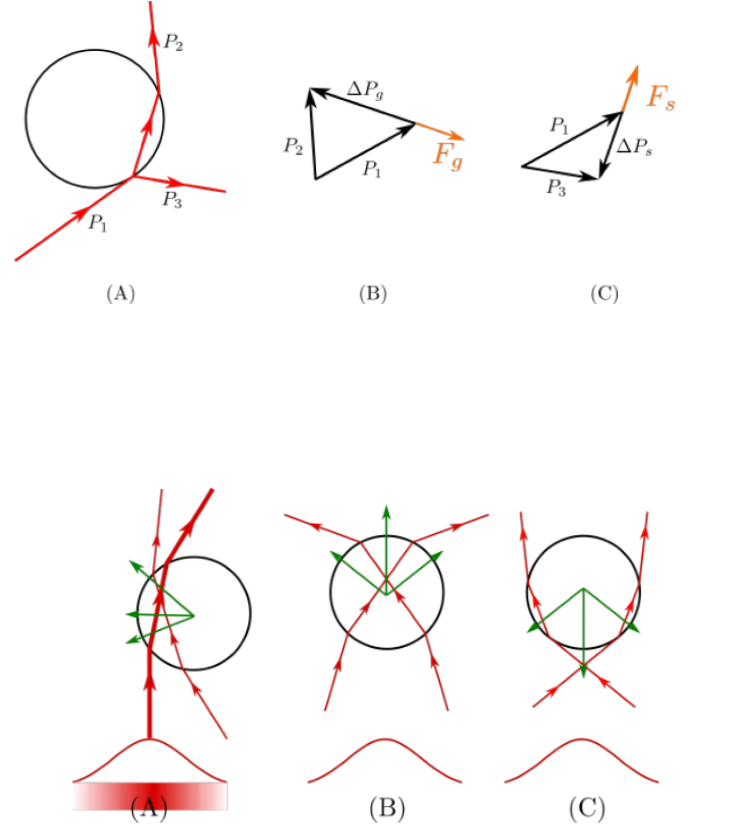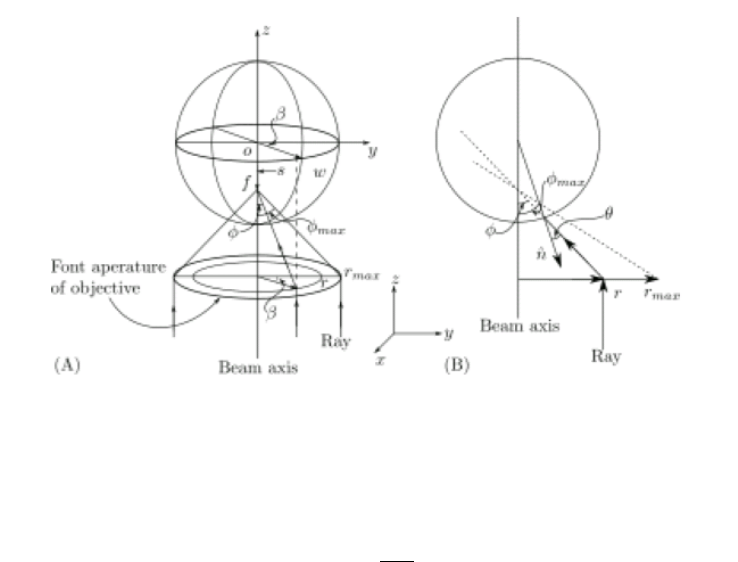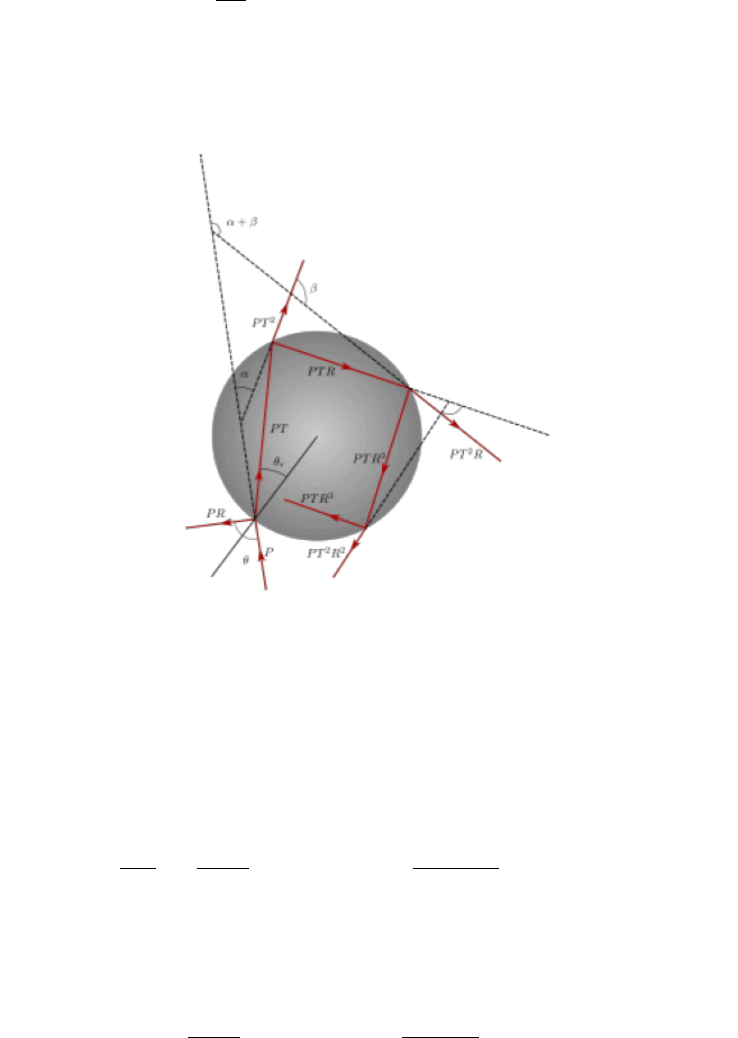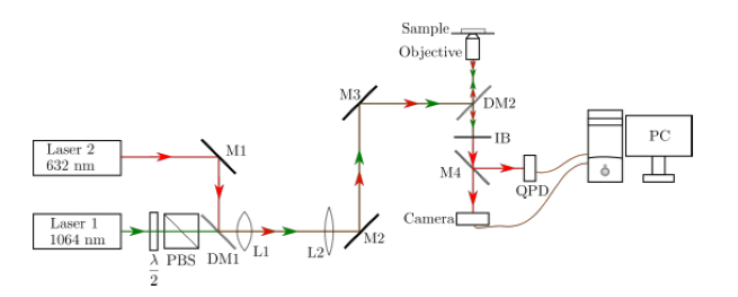Summer Research Fellowship Programme of India's Science Academies 2017Trapping of a Polysterene latex
beads using optical tweezers
Rama chaudhary
July 15, 2017
Certiﬁcate
This is to certify that the project entitled “Trapping of Polysterene Latex Bead
using Optical Tweezers” was carried out by Ms. Rama Chaudhary, Dayalbagh
Educational Institute at department of physical sciences at the Indian Institute
of Science, Bangalore under my guidance in the partial fulﬁllment of her summer
research fellowship. The duration of this project was from 22nd May, 2017 to
17thJuly, 2017.
Prof. Vasant Natarajan
Department of Physical Sciences
Indian Institute of Science
Bengaluru
Karnataka-560012
1
Declaration
I hereby declare that project report entitled “Trapping of Polysterene Latex
Bead using Optical Tweezers” is the authentic record of genuine work done
under the guidance of Prof. Vasant Natarajan at the department of Pjysical
Sciences, Indian Institute of Science, Bengaluru in partial fulﬁllment of my
summer research fellowship.
Date: 17th July, 2017
Place: Bengaluru
Rama Chaudhary
PHYS928
2
Acknowledgement
First of all I want to thank joint summer research fellowship programme which
provided me this golden opportunity to work as a summer fellow. I want to
thank my guide Prof. Vasant Natarajan, who constantly adviced me and guided
me to a meaningful conclusion. I would acknowledge him for his valuable role
in completion of my internship.I want to thank Sudheer Anand S. whom Prof.
Vasant has assigned as a co-guide for me, for his continous help and advice. I
want to thank all Lab members for maintaining such a cheerful and positive
environment. I would acknowledge Indian Academy of sciences for providing
food and residence, which was really comfortable. I would also be greatful to
my teacher Prof. Sukhdev Roy, who recommend me for this internship. In the
last I want to thanks my family for always being with me and all summer fellows
staying in academy guest house for giving me laot of memmories and support.
Rama Chaudhary
M.Sc Physics
Dayalbagh Educational Institute
Agra- U.P.
3
Contents
0.1 Introduction . . . . . . . . . . . . . . . . . . . . . . . . . . . . . . 6
0.1.1 Basic Forces involved in trapping . . . . . . . . . . . . . . 6
0.1.2 Theory of trapping . . . . . . . . . . . . . . . . . . . . . . 7
0.1.3 Applications of Optical tweezers . . . . . . . . . . . . . . 12
0.2 Experimental Setup . . . . . . . . . . . . . . . . . . . . . . . . . 12
0.2.1 Various components of appratus used . . . . . . . . . . . 12
0.2.2 Quadrant Photo Detector: . . . . . . . . . . . . . . . . . . 15
0.3 Results . . . . . . . . . . . . . . . . . . . . . . . . . . . . . . . . . 16
0.4 Conclusion . . . . . . . . . . . . . . . . . . . . . . . . . . . . . . 19
4
List of Figures
1 (A)Path of light after reﬂection and refraction from the micro-
scopic particle (B)Change of momentum due to refraction of light
which generates gradient force (C) Change of momentum due to
reﬂection of light which generates scattering force . . . . . . . . . 8
2 Diagram shows forces for diﬀerent positions of bead compared to
the focus of the beam (A) when particle is horizontally oﬀ-center
and vertically at same level of focus (B) when the bead is above
focus (C) when the bead is below focus . . . . . . . . . . . . . . 8
3 (A) Three dimensional view of a view of a single beam gradient
force in the geometrical optics approach. (B) The two dimen-
sional view of the same. . . . . . . . . . . . . . . . . . . . . . . . 9
4 Consecutive reﬂection and refraction of a single ray inside the
spherical particle . . . . . . . . . . . . . . . . . . . . . . . . . . . 10
5 Schematic diagram for optical tweezers setup used . . . . . . . . 12
6 This side contains lasers, PBS, waveplate, mirrors and lenses . . 13
7 This side contains periscope and microscope. . . . . . . . . . . . 13
8 Woring principle of piezomotor (A) stationary state (B) when
stage move slowly screw rotate because of staticfriction. (C)when
it rotate fast stage sleep because of inertia and keep rotatng in
one direction. . . . . . . . . . . . . . . . . . . . . . . . . . . . . . 15
9 We can detect the movement of beaad using movement of spot
on QPD . . . . . . . . . . . . . . . . . . . . . . . . . . . . . . . . 15
10 Circuit diagram of quadrant photodetector . . . . . . . . . . . . 16
11 Untrapped bead . . . . . . . . . . . . . . . . . . . . . . . . . . . 17
12 Bead got trapped when it reached near to beam . . . . . . . . . . 17
13 Bead remains trapped even the stage is moving . . . . . . . . . . 18
14 Bead remains trapped even the stage is moving . . . . . . . . . . 18
50.1 Introduction
Optical tweezers is the instrument which uses the momentum of light to apply
force on a microscopic particle and hence it can be used to trap it. The forces
applied by this instrument can be very less to order of pico-newton. These
small forces are very useful in the ﬁeld of biophysics, especially in the study of
biological cells. This instrument leads to non-invasive techniques of study and
manipulation of biological cells i.e. manipulation without any damage. That is
why popularity of optical tweezers increased very rapidly in last few decades.
The presence of radiation pressure on small particles was ﬁrst detected ex-
perimentally by Nicholas and Hull  in the beginning of 20th century but it
was invention of lasers which enable A. Ashkin to show that this force may
be used to control the dynamics of small neutral particles. He showed that,
particles can be accelerated, decelerated or stably trapped using these radiation
forces. This trap means particle forms a stable equilibrium point in space and
if a force try to deviate particle from its equilibrium position then a restoring
force always bring it back towards it.
The basic principle behind trapping is radiation pressure of light. For a
perfectly reﬂecting mirror,change in radiation pressure per second and hence
force by an incident beam of power P is given by
2P
c
,where c is velocity of
light. So, light beam of 1W exerts a force of 7nW of surface, which is very
small and of no use. But because of lasers one can, now, achieve a tightly
focused beam. Due to small size and mass of particle the radiation pressure of
1W laser is, now, 10
5
times the gravitational force on the particle. This force
is large enough to aﬀect the dyamics of the particle.
Nowdays, These techniques of optically trapping and manipulation are very
popular in the ﬁelds where dynamics of single particle is involved. The ﬁeld
of molecular chemistry, superconductivity, cold atom physics and many more
get their beneﬁts from these techniques. The force is small so biological cells
and living organisms can also be trapped and manipulated without fear of any
damage. That is why, these techniques have huge advantage in ﬁeld of biophysics
and biology. infact at present, I am working on a apparatus which was used by
Apurba Paul to study healthy and infected red blood cells to detect malaria. As
we shall see, these techniques are simple and very advantageous in many ﬁelds.
0.1.1 Basic Forces involved in trapping
In beginning, A. Ashkin only seeked to accelerate a particle using that radiation
forces considering it is perfectily reﬂecting. He consider that a single photon
has momentem
c
and if P is power of incident beam than total change in
momentum will be twice the number of photons multiply by momentum of
single photon, which turn out to be
2P
c
, as I stated before. Using a laser tightly
focused in small spot of micrometer range and let it hit a particle of same size as
spot we can get acceleration 10
5
g, where g is acceleration due to gravity of earth.
He designed a experiment and succeded to get accelaration approximately equal
to his estimates.This proved that trapping is a radiation pressure phenomena.
6
But during this experiment another unrecognized force came into act which
always tries to pull particle towards the point of highest intesity. Once the
particle reaches the center of the beam i.e. point of highest intensity(because
Our experiment involve a gaussian T EM
00
beam ), it stays there even if we
move the beam up and down and in any direction. This force is gradient force
which is responsible for trapping of bead, while, ﬁrst force is known as scattering
force which is in direction of propogation of light beam and destablize the trap.
For trapping to occur gradient force must always be greater than the scattering
force , which is only possible if refractive index of particle is greater then that
of medium.
0.1.2 Theory of trapping
The trapping depends on diﬀerent kinds of factor other than like shape and
size of particles, power of laser, power and numerical aperture of the objective.
it is possible to trap diﬀerent sizes of particle ranging from nanometers to mi-
crometers, but to explain it theortically we have to devide whole range in two
regime.
Mie Regime - When the particles diameter is much larger than the wave-
length of the trapping beam.
Rayleigh Regime - When the particle diameter is much smaller than the
wavelength of trapping beam.
Since in our case, particle diameter d = 3 microns is much larger than the
wavelength of trapping beam λ = 1.064 microns I will only discuss Mie regime
theory. In this regime, we can use ray optics approach because diﬀraction
is neglisible. the trapping in this regime can be explained qualitatively by
refraction and reﬂection of beam incident on the bead.
Let us consider that beam incident on spherical bead has a momentum
~
P
1
,
beam leaving the sphere has momentum
~
P
2
and beam reﬂecting from the surface
has a momentum
~
P
3
ﬁgure 1(A). Now, momentum transfer to the bead due
to refraction will be
~
P
g
=
~
P
2
~
P
1
as shown in ﬁgure 1(B). The force due
to this presssure
~
F
g
is gradient force and will be in direction opposite to the
propogation of light. The momentum transfer to the bead due to reﬂection will
be ∆
~
P
s
=
~
P
3
~
P
1
ﬁgure 1(C). This pressure will generate scattering force
~
F
s
.
Figure 2 demonstrate that if particle is at the center of the beam than no
horizontal force will act on it and it will remain in the center.
7Figure 1: (A)Path of light after reﬂection and refraction from the microscopic
particle (B)Change of momentum due to refraction of light which generates gra-
dient force (C) Change of momentum due to reﬂection of light which generates
scattering force
Figure 2: Diagram shows forces for diﬀerent positions of bead compared to the
focus of the beam (A) when particle is horizontally oﬀ-center and vertically at
same level of focus (B) when the bead is above focus (C) when the bead is below
focus
whereas, if it is oﬀ center than the light which comes out from the center of
the beam will be more intense than light coming out from edges of the beam.
Hence there will be a horizontal force which will pull bead towards center and
hence the bead will be horizontally trapped.
Figure 2(B) and 2(C) show that if bead moves below and above of focus
8of the beam then gradient force will pull it up and down respectively. Which
again proves that gradient force is always directed towards the focus of Gaussian
beam.
To calculate the force on the particle by light theoritically, we can calcu-
late the force by a single ray and then sum up all the contributing rays. Let
us consider then center of bead is at the axis of the beam. The distance of
considered ray from axis of beam is r and it makes an angle β with y-axis. as
shown in ﬁgure 3. we can calculate the force for a single beam and to get the
total force , we integrate it to whole range of r i.e 0 to r
max
from axis of sphere,
and for whole range of β with respect to the y-axis. As described by Wright et.
al. we can use the T EM
00
Gaussian mode of beam propogation to get the
direction of the ray and angle θ with the respect to the normal of the surface of
the microsphere. Although there are some ﬂaws in model.
Figure 3: (A) Three dimensional view of a view of a single beam gradient force
in the geometrical optics approach. (B) The two dimensional view of the same.
The radius of curvature of the beam wave front is given by r
R(z) = z
1 +
πω
2
0
λz
2
!
(1)
where ω
0
is the spot size at the focus of the laser beam at the medium. This
formula tells that radius of curvature vary as wave propogate i.e. a beam can
bend even in single refractive index medium, which is not possbile in ray optics
approach. so, contradiction arises to our assumptions. For good trap divergence
of beam should be high. But high divergence leads to high bending. Even with
these ﬂaws our model is close enough to ray optic model.
9Consider a single ray of power P hitting the sphere. The momentum per
second is thus given by
n
2
P
c
where n
2
is refractive index of the medium. The
force on the sphere will be the sum of the contributions from the reﬂected ray
with power P R and the inﬁnite number of rays coming out from the sphere with
powers P T
2
, P T
2
R, P T
2
R
2
...P T
2
R
n
...Here R and T are fresnel reﬂection and
trasmission coeﬃcient for the surface of the particle at the media at incident
angle θ, see ﬁgure4.
Figure 4: Consecutive reﬂection and refraction of a single ray inside the spherical
particle .
The reﬂected and scattered ray emerging from the sphere are making angle
π + 2θ, α, α + β, α + 2β...α + ...respectively. Now the total force in the
z-direction is the net change of momentum along z direction per second , which
is given by
F
z
=
n
2
P
c
"
n
2
P R
c
cos(π + 2θ) +
X
n=0
n
2
P T
2
R
n
c
cos(α + )
#
(2)
Since ﬁrst term on Right hand side is momentum of incident. Similarly, force
in y-direction will be total momentum change in y- direction per second. initial
momentum in y-direction was zero. So we get
F
y
= 0
"
n
2
P R
c
sin(π + 2θ)
X
n=0
n
2
P T R
n
c
sin(α + )
#
(3)
10The forces F
s
and F
g
are independent so they can be combined in a complex
plane to get total force, F
total
= F
z
+ iF
y
.
F
total
=
n
2
P
c
(1 + R cos θ) + i
n
2
P
c
R sin 2θ
n
2
P
c
T
2
X
n=0
R
n
e
i(α+)
(4)
clearly, last term on right hand side consist a geometric progression term. We
can easily ﬁnd the sum of this series, Hence we get
F
total
=
n
2
P
c
(1 + R cos θ) + i
n
2
P
c
R sin 2θ
n
2
P
c
T
2
e
1
1 Re
(5)
Now, rewriting equation (5), we get
F
total
=
n
2
P
c
(1 + R cos θ) + i
n
2
P
c
R sin 2θ
n
2
P
c
T
2
e
1
1 r cos β + iR sin β
(6)
To make it simpler, we must rationalize the equation (6), i.e.
F
total
=
n
2
P
c
(1+R cos θ)+i
n
2
P
c
R sin 2θ
n
2
P
c
T
2
e
1 R cos β iR sin β
(1 r cos β)
2
+ R
2
sin
2
β
(7)
Solving and seperating real and imaginary terms, we get
Re(F
total
) =
n
2
P
c
(1 + R cos θ)
n
2
P T
2
c
cosα(1 R cos β) + R]sinα sin β
(1 R cos β)
2
+ R
2
sin
2
β
(8)
Im(F
total
) =
n
2
P R
c
sin 2θ
n
2
P T
2
c
sin α R sin α cos β R cos α cos β
(1 R cos β)
2
+ R
2
sin
2
β
(9)
We know that above two eqaution gives values for F
z
= F
s
and F
y
= F
g
respectively. Now, if we use the relations α = 2θ 2θ
r
and β = π 2θ
r
, where
θ and θ
r
are the angle of incidence and refraction respectively, then we get
F
s
=
n
2
P
c
1 + R cos θ
T
2
[cos(2θ 2θ
r
) + R cos 2θ]
1 + R
2
+ 2R cos 2θ
r
(10)
and,
F
g
=
n
2
P
c
R sin 2θ
T
2
[sin(2θ 2θ
r
) + R sin 2θ]
1 + R
2
+ 2R cos 2θ
r
(11)
These formulas are for single incoming ray. The force due to all refracted
rays for that single ray has been added. to get total force on the bead , one
have to take vector sum of forces applied by all individual rays. Since R and T
are polarization dependent, hence this force is also polarization dependent.
110.1.3 Applications of Optical tweezers
Optical tweezers has lead to many application like high resolution in Mie scat-
tering, high resolution study of resonant microscopic particles. In ﬁeld of atomic
physics laser trapping and cooling enable the study of Bose- Einstien conden-
sation and atom lasers. Present advances in atomic clocks and measurement of
gravitational force have also been made. As I mentioned before, they are very
beneﬁtial in biophysics and study of single biological cell.The use of optical
tweezer techniques has led to an explosion of new understanding of the me-
chanics, force generation, and kinetics of a wide variety of motormolecules and
mechanoenzymes.The unique ability of tweezers to manipulate small macro-
scopic particles has been used in colloidal science to detect anomalous new
eﬀects. There are many more applications of optical tweezers.
0.2 Experimental Setup
In a very basic optical tweezers setup, we need a high power continuous wave
laser source, a high numerical aperture objective to tightly focus laser beam,some
arrangement to precisely move the sample and an imaging system. schematic
diagram for setup used is given in ﬁgure 5 and picture of setup is given in ﬁgure
6 and ﬁgure 7.
Figure 5: Schematic diagram for optical tweezers setup used
0.2.1 Various components of appratus used
Lasers: In my experiment , I have used two continous wave laser. One is
Nd:YAG Diode pump solid state laser(LSR 1064L-500, LASERVER,China) hav-
ing wavelength 1064 nm, while other is He-Ne laser(MELLES GRIOT,China).
Nd:YAG bead is for trapping the bead. Since this laser is high power hence we
avoide its exposure to sensitive instruments. That is why red laser He-Ne (632
nm) is used to track the trapped bead.
12